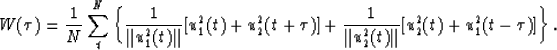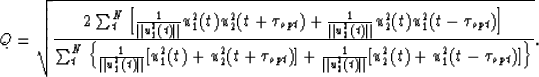Next: EXAMPLES Up: LINEAR OPTIMIZATION Previous: Objective function

## The quality of picking

As two neighboring traces will not always be matched perfectly just by shifting, it is useful to define some quantity that measures the quality of the picking process. This quantity will have its value between and 1. It will equal to when input is all-zero or white noise, equal to 1 when the matching can be made perfectly. In the plane-wave destructor method, Claerbout (1990) chose the normalized correlation of the partial derivatives of data as a measure. Here, we will use the normalized correlation of data itself:(10)
whereBy insertingin equation (3) into this definition, we have(11)

The computations of the linear optimization and the quality measure can be done in a similar way to that of the nonlinear optimization. The code vectorizes fully.Next: EXAMPLES Up: LINEAR OPTIMIZATION Previous: Objective function
Stanford Exploration Project
1/13/1998